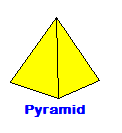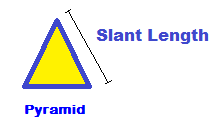CalcuNation

# Triangular Pyramid Surface Area Calculator

## Find the surface area of a pyramid with a triangular base with this Pyramid Surface Area Calculator.Base Perimeter: To find the perimeter of a triangle use:
Triangle Perimeter Calculator

Base Area: To find the area of the triangular base use:
Triangle Area Calculator

Slant Length: This is the distance from the center of the base on one
of the lateral surface triangles, to the tip of that surface triangle.

Fill in the perimeter and area of the triangular base of a regular pyramid,
and the height of the regular pyramid to determine surface area.

### How do you find the surface area of a pyramid?

To find the surface area, use this pyramid surface area formula:
Surface Area = ( .5 x Base Perimeter x S ) + Base Area

S = Slant length of one of the triangular facesExample: The surface area of a regular triangular pyramid with base area
of 32 square inches, base perimeter 24 inches and slant length of
8 inches:

Surface Area = ( .5 x 24 x 8) + 32

Calculated out this gives a surface area of 128 Square Inches.

### \$100 Promotion

Win \$100 towards teaching supplies! We want to see your websites and blogs.Enter Here

### Calculator Popups

Scientific Calculator
Simple Calculator

### Calculator Ideas

We use your calculator ideas to create new and useful online calculators.
Submit Calculator Idea